American Journal of Software Engineering and Applications
Volume 5, Issue 6, December 2016, Pages: 61-67

Development of Matlab-Based Software for the Design of the Electric Circuit of Three-Phase Power Transformer

Ezenugu Isaac A.1, Idorenyin Markson2, Bliss Utibe-Abasi Stephen3

1Department of Electrical Engineering, Imo State University (IMSU), Owerri, Nigeria

2Department of Mechanical Engineering, University of Uyo, Uyo, Nigeria

3Department of Electrical/Electronic and Computer Engineering, University of Uyo, Uyo, Nigeria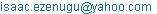(Ezenugu I. A.)

Ezenugu Isaac A., Idorenyin Markson, Bliss Utibe-Abasi Stephen. Development of Matlab-Based Software for the Design of the Electric Circuit of Three-Phase Power Transformer. American Journal of Software Engineering and Applications. Vol. 5, No. 6, 2016, pp. 61-67. doi: 10.11648/j.ajsea.20160506.14

Received: August 6, 2014; Accepted: January 4, 2017; Published: February 2, 2017

Abstract: Power transformers are crucial components in electric power systems, as such, it is required that they are built to highest standards of precision and quality and designed for a long life-time. As the average age of these transformers is in the range of 30 to 40 years in many countries, the probability of malfunction is on the rise. This situation is accentuated by the trend of operating transformers closer to their performance limits, adding to their vulnerability if adequate countermeasures are not taken. In this paper, MATLAB-based software was developed for automatic computation of the electric circuit parameters of a three phase power transformer once the input specifications are supplied. A sample design problem was used to demonstrate the effectiveness of the program. The automated computation program was developed using the mathematical models derived for the various parameters to be determined. Apart from its flexibility and speed, the program removed the drudgery involved in the design. In addition, the MATLAB-based software presented in this paper will serve as a useful teaching and laboratory tool for undergraduate courses in transformer design.

Keywords: Transformer, Electric Circuit, Power Transformer, Three Phase, Single Phase, MATLAB

1. Introduction

Over the years, transformers have been used extensively in electric power systems to transfer power by electromagnetic induction between circuits at the same frequency, usually with changed values of voltage and current . Given its importance, transformer design is a big business in the electric power industry. Basically, the aim of transformer design is to obtain the dimensions of all parts of the transformer in order to supply these data to the manufacturer. However, the complexity of transformer design demands reliable and rigorous solution methods. Transformer design is a complex task in which engineers have to ensure that compatibility with the imposed specifications is met, while keeping manufacturing costs low ,  Indeed, it is difficult if at all possible, to meet accurately today’s transformer electrical parameters via conventional design techniques . In view of the challenges, an alternative, user-friendly and effective way for calculating the transformer parameters through the use of software is seriously required. Given that MATLAB is one of the most popular mathematical programs used in engineering analysis, in this paper a MATLAB–based software tool will be developed for the design of the electric circuit of power transformers. In this case, the software tool will make use of the MATLAB Application Program Interface (API) to extend the functionalities of MATLAB application to include the design of the electric circuit of power transformers.

Specifically, this paper presents the design of the electric circuit of power transformers using the MATLAB–based software presented in this paper. Sample design problem is used to demonstrate the effectiveness of the software solution.

2. Review of Related Works

A transformer has been defined by ANSI/IEEE  as a static electric device consisting of a winding, or two or more coupled windings, with or without a magnetic core, for introducing mutual coupling between electric circuits . Transformers operation depends on electromagnetic induction between two stationary coils (the electric circuit) and a magnetic flux of changing magnitude and polarity (the magnetic circuit). In essence, transformers transform electrical energy into magnetic energy, and then back into electrical energy.

Given its importance, transformer design is a big business in the electric power industry. Basically, the aim of transformer design is to obtain the dimensions of all parts of the transformer in order to supply these data to the manufacturer. The transformer design should be carried out based on the specification given, using available materials economically in order to achieve low cost, low weight, small size and good operating performance. The transformer design is worked out using various methods based on accumulated experience realized in different formulas, equations, tables and charts . Transformer design is a complex task in which engineers have to ensure that compatibility with the imposed specifications is met, while keeping manufacturing costs low [1-2] In addition, in order to compete successfully in the global economy, transformer manufacturers need design software capable of producing manufacturable and optimal designs in a very short time. Over the years, several design procedures for transformers have appeared in many literatures [6-8]. Some of the literatures are targeted at transformer design for teaching and hands-on training purposes [8-10]. Furthermore, other literatures presented the development or the use of various computer programs for transformer design , [11-13].

Specifically, this paper presents the design of the electric circuits of power transformer and the implementation of sample design problem using the MATLAB–based software.

3. Methodology

First mathematical expressions for computing the values of various parameters of the electric circuit of power transformer are derived based on the assumptions that certain design specifications are given. Secondly, the algorithm for automating the computation of those parameters with the use of computer software is developed. Thirdly, MATLAB-based software was developed to read in design specifications as input and then automatically compute and display the various parameters of the electric circuit of power transformer.

The development of the MATLAB software for designing the electric circuit of power transformers consists of the following:

the design of different graphical user interfaces (GUIs) for entering the design input parameters

the design of program to automatically compute and display the remaining parameters of the electric circuit of power transformer based on the mathematical expressions presented in this paper

aggregating the various GUIs and computation modules into a unified standalone application using the MATLAB deployment tool.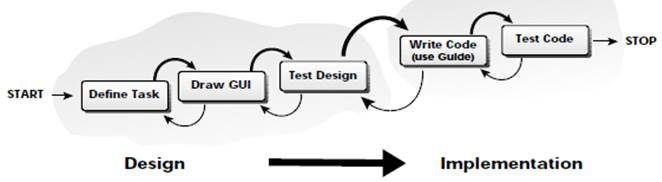Figure 1. GUI Design and Testing.

In all, the design and implementation of the MATLAB software was done using the MATLAB’s GUI development environment, also known as the M-GUIDE. The methodology used for the design and testing of the GUI is given in Figure 1.

3.1. Design Specification

The transformer design begins with the specifications of the transformer to be designed. The specifications give the rating and performance expectation of the transformer. Some useful specifications for the design of electric circuit of power transformer include;

1. Transformer Power rating, S in KVA

2. Frequency, f in Hz

3. Line voltage of the high voltage (HV) winding, Vinhv

4. Connection type – star or Delta

5. Percentage impedance, Z%

6. Tapping on the H. V winding, Tp1, Tp2

Furthermore, in the electric circuit design, it is assumed that some parameters pertaining to the electric circuit of the transformer have been obtained or are give as part of the design specifications; among these parameter are voltage per turn (Vt) iron core cross sectional area (Ai), the window height (Hw), overall core frame height, (H) overall core frame width (W) and diameter of the core limb (Dsc).

3.2. Winding Design

The winding consist of the Low Voltage (L. V) and high Voltage (H. V) windings. This paper will consider only disc winding with rectangular conductor.

3.2.1. Low Voltage (L. V) Winding Design

1. Phase voltage (Vplv):

For star connection Vplv=Vlnlv/√3        (1)

For delta connection Vplv=Vlnlv         (2)

Where Vlnlv is the line voltage for the low side of the transformer

2. Number of turn

(Nlv): Nlv=Vplv/Vt              (3)

Where Vt is the voltage per turns

3. Phase current (Iplv):

For star connection Iplv=S/ (√3 Vplv)      (4)

For delta connection Iplv=S/ (3 Vplv)      (5)

4. Phase current area of the conductor (L. V), alv

alv=Iplv/ Jid (Choose current density Jid in A/mm2)  (6)

5. L. V conductor dimensions

The conductor parameters are obtained as follows;

(Bare conductor width, Wbcdlv bare conductor thickness tbcslv, number of conductors per turn, nptlv, conductor insulator thickness tins)

Area of the bare conductor,

abcdlv=(Wbcdlv) (tbcslv) (nptlv)           (7)

With the insulation on the conductor, dimension becomes

Insulated conductor width,

Wicdlv=Wbcdlv+tins               (8)

Insulated conductor thickness, ticdlv:

ticdlv=tbcslv+tins cc                (9)

Area of insulated conductor, aicdlv:

aicdlv=Wicdlv (ticdlv) (nptlv)           (10)

Since the dimension of the conductor are chosen, the area of the conductor, abcdlv may be different from the area, alv computed earlier, hence, the current density will change. The actual current density is given as

Jic=Iplv/abcdlv                  (11)

6. Disc winding of L. V winding

Choose the number of disc (or coil) ndclv

The number of turn per disc,

ntdclv=Nlv/ndclv                 (12)

Choose the number of layers per disc, nlylv

Number of turns per layer per disc, ntlylv:

ntlylv=ntdclv/ nlylv               (13)

The dimensions of each turn of the coil are as follows;

Width of each turn of coil

Wtclv=Wicdlv                (14)

Thickness of each turn of coil,

ttclv=ticdlv×nptlv               (15)

Height of coil,

Hpclv=ntlylv×Wtclv             (16)

Thickness of coil,

tpclv=ntlylv×ttclv              (17)

Choose the distance between adjacent coils or disc, dbclv

Height of low voltage winding,

Hndglv=(Hpclv×ndclv)+(ndelv-1) ddelv     (18)

Thickness of low voltage winding,

tndglv=tdclv                (19)

7. Diameter and Length of Low Voltage Winding

Choose the distance core and L. V winding, ddclv

The inside diameter or L. V coil, dilv=core diameter+2 (dclv)

Dilv=Dsc+2 (dclv)             (20)

Outside diameter of L. V coil (dolv):

dolv=dilv+2 (tndgv)            (21)

Mean diameter of L. V winding (dmlv):

dmlv=dilv+tndglv             (22)

Mean length of L. V winding Lmlv:

Lmlv=π (dmlv)              (23)

3.2.2. High Voltage Winding Design

1. Phase voltage (Vphv):

For star connection Vphv=Vlnhv/√3       (24)

For delta connection Vphv=Vlnhv        (25)

Where Vlnhv is the line voltage of the high voltage side of the transformer

2. Number of turns (Nhv):

Nhv=Vphv/V                (26)

3. Phase current (Iphv): for star connection,

Iphv=S/(√3Vphv)               (27)

For delta connection,

Iphv=S/(3Vphv)               (28)

4. Cross sectional area of the conductor (ahv):

anv=Iphv / Jid                (29)

5. Conductor dimension: The conductor used is rectangular, choose the appropriate conductor dimensions and number of conductors. The conductor parameters are as follows, bare conductor width, Wbcdhv, bar conductor thickness, tbcdhv, number of conductor stripes per turn, npthv,

Area of bare conductor, abcdhv:

abcdhv=Wbcdhv×tbcdhv×npthv        (30)

Choose the conductor and hence the insulator thickness, tins. With the insulation on the conductor, the dimensions become:

Insulated conductor width, Wicdhv:

Wicdhv=Wbcdhv+tins           (31)

Insulated conductor thickness, ticdhv:

ticdhv=tbcdhv+tins            (32)

Area of insulated conductor aicdhv:

aicdhv=ticdhv×npthv           (33)

6. Disc winding design for the H. V Winding

Choose the number of disc (coil) ndchv,

The number of turn per disc, ntdchv:

ntdchv=Nhv/ ndchv            (34)

Choose the number of layers per disc, nlyhv

The number of turns per layer per disc, ntlyhv:

ntlyhv=ntdchv/nlyhv(35)

The dimensions of the each turn of the coil are as follows

Width of each turn of coil, Wtchv=Wicdhv(36)

Thickness of each coil, ttchv=ticdhv×npthv   (37)

Height of coil, Hpchv=ntLyhv×Wtchv     (38)

Thickness of coil tpchv=nlyhv×ttchv     (39)

Choose the distance between adjacent coils (or discs) dbchv

Height of high voltage winding, Hndghv:

Hndghv=(Hpchv×ndchv)+(ndchv-1) dbchv    (40)

Thickness of high voltage winding, tndghv:

tndghv=tpchv                (41)

7. Diameter and length of high voltage winding

Choose the distance between the low voltage winding and the high voltage winding, dlvhv

Inside diameter of high voltage winding,

dihv=dolv+2 (dlvhv)            (42)

Outside diameter of high voltage winding, dohv:

dohv=dilv+2 (tndghv)            (43)

Mean diameter of high voltage winding, dmhv:

dmhv=dohv+tndghv            (44)

Mean length of H. V coil, Lmhv:

Lmhv=πdmhv                   (45)

3.3. Percentage Reactance (Xpct) and Percentage Resistance (Rpct)

Average length of turn Lmt:

Lmt=(Lmlv+Lmhv)/2             (46)

Ampere turn,

Atn=Iplv×Nlv               (47)

Mean height of turn, Hmt:

Hmt=(Hndglv+Hndghv)/2         (48)

Percentage resistance, Xpet:

Xpet=Atn (2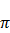0Lmt) (dlvhv+(tndglv+tndghv))(49)

Where, µ0=4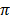×10-7

ρ20=0.01724 ohm/mm2/m, α20=0.00 393

At the temperature of Tmp°C, ρTmp is given as

ρTmp20 (1+α20 (Tmp-20))        (50)

Resistance of the low voltage winding,

Rndglv=(ρTmp×Lmlv×Nlv)/ (alv×1000)     (51)

Resistance of the high voltage winding,

Rndghv=(ρTmp×Lmhv×Nhv)/ (alv×1000)    (52)

Ratio of transformation,

khlv=Vphv/Vplv             (53)

Equivalent resistance referred to high voltage side,

Rrhv=Rndghv+Rndglv (Khlv)2         (54)

Percentage resistance,

Rpct=(Iphv×Rrhv)/ Vphv        (55)

3.4. Percentage Impedance (Zpct) and Core Loss

Zpct=√ ((Xpct)2+(Rpct)2)        (56)

Volume of iron, Vi=Ai (2W+3H)   (57)

Where Ai is cross sectional area of iron, H is the overall core frame height, W is the overall core frame width and Ai, W and H are given in the design specification

Weight of iron, Wi=(Vi×ρi)       (58)

where density of iron, ρi is given as

Choose core loss per kilogram of iron (KLkg), KLkg

Core loss Lcor=Wi×KLkg         (59)

3.5. Weight of Copper and Copper Loss, Lcpp

Read Magnetizing Volt-ampere per kilogram (Kvakg)

Magnetizing volt-ampere, MVA

MVA=Wi×(Kvakg)             (60)

Weight of L. V winding

Wlvcp=(ρcp×Nlv×LmLV aLV)/ (100x1000)    (61)

Where ρcp is the density of copper is given as

Weight of L. V winding

Whvcp=(ρcp×Nhv×Lmhv aHv)/ (100x1000)   (62)

Total weight of copper,

Wthlcp=3 (Whvcp+Wivcp)            (63)

Lcpp=3 (Rrhv) (Iphv)2              (64)

Lcpp=1.07 (3 (Rrhv) (Iphv)2)          (65)

Total Loss, Licp is given as;

Licp=Lcor+Lcpp                  (66)

3.6. Efficiency and Regulation at Unity Power Factor

1. Efficiency on full load, Eff1:

Eff1=(Sx103)/(Sx103)+Licp)100%        (67)

2. Efficiency at ¾ full load at unity power factor, Eff2

Copper loss at ¾ full load:

Lcpp3/4=(3/4)2×Lcpp              (68)

Total loss at ¾ full load:

Licp3/4=Lcor+Lcpp3/4              (69)

Eff2=(((3/4) Sx103 / ((3/4) (Sx103)+Lcpp3/4)) 100%  (70)

3. Efficiency at ½ full load at unity power factor, Eff3

Copper loss at ½ full load

Lcpp1/2=(1/2)2×Lcpp             (71)

Total loss at ½ full load,

Licp1/2=Lcor+Lcpp1/2             (72)

Eff3=(((1/2) Sx103)/(1/2) (Sx103)+Lcpp1/2))100%  (73)

1. Regulation at unity power factor, Reg1

E=√ ((1+(Rpct/100)2+(Xpct/100)2)       (74)

Thus,

Reg1=(E – 1) 100%             (75)

2. Regulation at 0.8 power factor, Reg0.8

Reg0.8=0.8Rpct+0.6Xpct            (76)

3.7. Other Parameters

Core Loss Current, (ICI) is given as;

Ici=Lcor / (3Vphv)              (77)

Magnetizing Current, IMI is given as;

Imi=MVA/ (3Vphv)             (78)

No Load Current, I0I is given as;

I0i=√ (Imi2+Ici2)             (79)

Tapping on the High Voltage Side is given as;

The tapping are given as percentage of the H. V winding, example tp1%, tp2%, tp3% then the number of turns for each of the tapping are given as Nphv1, Nphv2, Nphv3, where

Nphv1=Nphv×(tp1/100)

Nphv2=Nphv×(tp2/100)

Nphv3=Nphv×(tp3/100)            (80)

4. Algorithm for Designing the Electric Circuit of 3-phase Power Transformer

4.1. Design Specifications

Obtain the values of the following parameters from the given design specifications

1. Power rating, S in KVA

2. Frequency, f in Hz

3. Line voltage of the high voltage (H. V) winding, Vinhv

4. Line voltage of the low voltage (L. V) winding, Vinlv

5. Connection types –delta or star

6. Percentage impedance

7. Tapping on the H. V winding

4.2. Low Voltage (L. V) Winding Design (Disc Winding)

4.2.1. L. V Voltage and Current Parameters

1. Compute L. V phase voltage, Vplv using Vplv=Vinlv/√3 for star connection or Vplv=Vinlv for delta connection

2. Compute L. V phase current, Iplv using Iplv=S/ (√3 Vplv) for star or Iplv=S/ (3 Vplv) for delta connection

4.2.2. Low Voltage Conductor Parameter

1. Compute the number of turns in L. V winding, Nlv form Nlv=Vplv/Vt

2. Choose current density, Jid

3. Compute cross sectional area of L. V conductor alv from alv=Iplv/ Jid

4. The winding type is disc and conductor is rectangular. look at the standard wire gauge table and choose cable stripe that can handle the L. V phase current Iplv. If one stripe cannot handle it then two or more conductors stripe can be chosen and combined where nptlv is the number of conductor so chosen from the table, read the width of the bare conductor stripe Wbcdlv and the thickness of the conductors, tbcdlv

5. Compute the area of the bare conductor stripe abcdlv from abcdlv=(Wbcdlv) (tbcdlv) (nptlv)

6. Choose conductor insulator thickness, tins

7. Compute insulated conductor width, Wicdlv from Wicdlv=Wbcdlv+tins

8. Compute insulate conductor thickness, ticdlv from ticdlv=tbcdlv+tins

9. Compute insulated conductor area, aicdlv from aicdlv=Wicdlv (ticdlv) (nptlv)

10. Compute actual current density Jic from Jic=Iplv/abcdlv

4.2.3. L. V Disc Winding Parameter

1. Choose number of disc, ndclv

2. Compute number of turns per disc, ntdclv from ntdclv=Nlv/ndclv

3. Choose number of layers per disc, nlylv

4. Compute number of layers per disc, ntlylv from ntlylv=ntdclv/ nlylv

5. Compute width of each turn of coil, Wtclv from Wtclv=Wicdlv

6. Compute thickness of each turn of coil ttclv from ttclv=ticdlv×nptlv

7. Compute height of each coil, Hpclv from Hpclv=ntlylv×Wtclv

8. Compute thickness of each coil, tpclv from tpclv=nlylv×ttclv

9. Choose the distance between adjacent coil, or disc, dbclv

10. Compute height of L. V winding, Hndglv from Hndglv=(Hpclv×ndclv)+(ndclv-1) diclv

11. Compute thickness of L. V winding, tndglv from tndglv=tdclv

12. Choose the distance between core limb and L. V winding, dclv

13. Compute inside diameter of L. V coil, dilv from dilv=Dsc+2 (dclv)

14. Compute inside diameter of L. V coil, dolv from dolv=dilv+2 (tndgv)

15. Compute mean diameter of L. V winding, dmlv from dmlv=dolv+tndglv

16. Compute mean length of L. V winding Lmlv from Lmlv=π (dmlv)

4.2.4. High Voltage Winding Design

1. Compute H. V phase voltage, Vphv from Vphv=Vlnhv/√3 for star connection or Vphv=Vlnhv for delta connection

2. Compute H. V phase current Iphv from Iphv=S/(√3Vphv) for star and Iphv=S/(3Vphv) for delta

4.2.5. High Voltage Conductor Parameter

1. Compute the number of turns in H. V winding, Nhn from Nhv=Vphv/V

2. Compute cross sectional area of H. V conductor ahv from ahv=Iphv / Jid

From the standard wire gauge table choose appropriate size of conductor and number of conductor stripe that can handle the given current Iphv. Let npthv be the choose number of conductor strips. Then read the width of the bare conductor stripe, wbcdlv read the thickness of the bare conductor stripe, tbcdhv

3. Compute area of bare conductor, abcdhvb from abcdhv=Wbcdhv×tbcdhv×npthv

4. Choose the conductor insulator thickness, tins

5. Compute width of insulated conductor, Wicdhv from Wicdhv=Wbcdhv+tins

6. Compute thickness of insulated conductor, ticdhv from ticdhv=tbcdhv+tins

7. Compute area of insulated conductor, aicdhv from aicdhv=Wicdhv×ticdhv×npthv

4.2.6. H. V Disc Winding Parameter

1. Choose number of disc, ndchv

2. Compute number of turns per disc, ntdchv from ntdchv=Nhv/ ndchv

3. Choose number of layers per disc, nlyhv

4. Compute number of turns per disc, ntlyhv from ntlyhv=ntdchv/nlyhv

5. Compute width of each turn of coil, Wtchv from Wtchv=Wicdhv

6. Compute thickness of each turn of coil, ttchv from ttchv=ticdhv×npthv

7. Compute height of coil, Hpchv from Hpchv=ntlyhv×Wtchv

8. Compute thickness of coil, tpchv from tpchv=nlyhv×ttchv

9. Choose the distance between adjacent coil (or disc), dbchv

10. Compute height of H. V winding, Hndghv from Hndghv=(Hpchv×ndchv)+(ndchv-1) dbchv

11. Compute thickness of winding, tndghv from tndghv=tpchv

12. Choose the distance between H. V winding and the H. N winding, dlvhv

13. Compute inside diameter of H. V winding, dihv from dihv=dsc+2 (dlvhv)

14. Compute inside diameter of H. V winding, dohv dohv=dilv+2 (tndghv)

15. Compute mean diameter of H. V winding, dmhv from dmhv=dohv+tndghv

16. Compute mean length of H. V coil, Lmhv from Lmhv=πdmhv

4.3. Percentage Impedance

1. Compute average length of turn, Lmt from Lmt=(Lmlv+Lmhv)/2

2. Compute ampere turn, Atn from Atn=Iplv×Nlv

3. Compute mean height of turn, Hmt from Hmt=(Hndglv+Hndghv)/2

4. Compute percentage reactance, Xpet from Xpet=Atn (2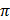0Lmt) (dlvhv+(tndglv+tndghv))

5. Compute resistance of L. V winding, Rndglv from Rndglv=(ρTmp×Lmlv×Nlv)/ (alv×1000)

6. Compute resistance of H. V winding, Rndghv from Rndghv=(ρTmp×Lmhv×Nhv)/ (alv×1000)

7. Compute ratio of transformation, Khlv from khlv=Vphv/Vplv

8. Compute equivalent resistance referred to H. V side, Rrhv from Rrhv=Rndghv+Rndglv (Khlv)2

9. Compute percentage impedance Zpct from Zpct=√ ((Xpet)2+(Rpet)2)

4.4. Transformer Losses

1. Compute volume of iron, Vi from Vi=Ai (2W+3H)

2. Compute weight of iron, Wi from Wi=(Vi×ρi)

3. Compute core loss, Lcor from Lcor=Wi×KLkg

4. Compute magnetizing volt ampere, MVA from MVA=Wi (Kvakg)

5. Compute weight of L. V winding, Wlvcp from Wlvcp=(ρcp×Nlv×LmLV aLV)/ (100x1000)

6. Compute weight H. V winding, Whvcp from Whvcp=(ρcp×Nhv×Lmhv aHv)/ (100x1000)

7. Compute total weight copper loss, Wthlcp from Wthlcp=3 (Whvcp+Wivcp)

8. Compute copper loss, Lcpp from Lcpp=3 (Rrhv) (Iphv)2

9. Compute total loss, Licp from Licp=Lcor+Lcpp

4.5. Efficiency of the Transformer

1. Compute efficiency at full load, Eff1 from Eff1=(Sx103)/(Sx103)+Licp)100%

2. Compute copper loss at ¾ full load, Lccp3/4 from Lcpp3/4=(3/4)2×Lcpp

3. Compute total loss at ¾ full load, Licp3/4 from Licp3/4=Lcor+Lcpp3/4

4. Compute efficiency at ¾ full load, Eff2 from Eff2=(((3/4) Sx103 / ((3/4) (Sx103)+Lcpp3/4)) 100%

5. Compute copper loss at ½ full load, Lcpp1/2 from Lcpp1/2=(1/2)2×Lcpp

6. Compute total loss at ½ full load, Licp1/2 from Licp1/2=Lcor+Lcpp1/2

7. Compute efficiency at ½ full load, Eff3 from Eff3=(((1/2) Sx103)/(1/2) (Sx103)+Lcpp1/2))100%

4.6. Regulation

1. Compute regulation at unity power factor, Reg1 from Reg1=(E – 1) 100%

2. Compute regulation at 0.8 power factor, Reg0.8 from Reg0.8=0.8Rpct+0.6Xpct

3. Compute core loss current Ici from Ici=Lcor / (3Vphv)

4. Compute magnetizing current Imi from Imi=MVA/ (3Vphv)

5. Compute no load current I0i from √ (Imi2+Ici2)

6. Compute tapping on H. V winding, Nphv1 from Nphv1=Nphv×(tp1/100) and Nphv2 from Nphv2=Nphv×(tp2/100)

5. Conclusion and Recommendation

5.1. Conclusion

In this paper, a standalone program was developed in MATLAB for the design of the electric circuit of a 3-phase power transformer. Mathematical models were developed for all the parameters to be computed and then a design algorithm was developed. Based on the mathematical models and algorithm the MATLAB-based software was developed and then a sample design problem was used to demonstrate how the program can be used. The ideas presented in the paper are extracts from undergraduate students’ hands-on projects. The simplicity of the mathematical models and the modular nature of the program make them relevant for teaching and hands-on training on power transformer design. It is also easy to upgrade the programs to accommodate the design of other kinds of transformers and the use of other design methodologies and also for incorporating optimization issues in the program.

5.2. Recommendation for Further Works

The scope of this paper covers only the design of the electric circuit of 3-phase power transformers. In particular, the design considered disc winding with rectangular conductors only. Further work is needed to cover other types of transformers and other form of winding layouts and conductor shapes. Also, design optimization was not considered in this paper. However, the modular nature of the program makes provision for the program to be easily upgraded to include the optimization module. Consequently, further work is required to develop the mathematical models and program codes for those additional modules.

References

1. Amoiralis, E. I., Tsili, M. A., & Georgilakis, P. S. (2008). The state of the art in engineering methods for transformer design and optimization: a survey. Journal of Optoelectronics and Advanced Materials, 10 (5), 1149.
2. Amoiralis, E. I., Tsili, M. A., & Kladas, A. G. (2009). Transformer design and optimization: a literature survey. Power Delivery, IEEE Transactions on, 24 (4), 1999-2024.
3. Georgilakis, P. S. (2009). Spotlight on modern transformer design. Springer.
4. IEEE (2002) IEEE Standard Terminology for Power and Distribution Transformers, IEEE Std C57.12. 80-2002.
5. Mittle VN, Mittal A (1996) Design of electrical machines, 4th edn. Standard Publishers Distributors, Nai Sarak, Delhi
6. Amoiralis. E. I., Georgilakis. P. S., Tsili. M. A., Kladas A.G.and Souflaris A. T. (2011) A complete software package for transformer design optimization and economic evaluation analysis. Materials Science Forum Vol. 670 (2011) pp 535-546) Trans Tech Publications, Switzerland
7. Judd F. F., Kressler D. R. (1977), IEEE Trans. Magn., vol. MAG-13, pp. 1058-1069.
8. Poloujadoff M., Findlay R. D. (1986), IEEE Trans. Power Sys., vol. PWRS-1.
9. Jewell W. T. (1990) IEEE Trans. Power Sys., vol. 5, pp. 499-505.
10. Grady W. M., Chan R., Samotyj M. J., Ferraro R. J., Bierschenk J. L. (1992) IEEE Trans. Power Sys.,vol. 7 (1992), pp. 709-717.
11. Rubaai A. (11994), IEEE Trans. Power Sys., vol. 9, pp. 1174-1181.
12. Andersen O. W(1991), IEEE Comput. Appl. Power, vol. 4, pp. 11–15.
13. Hernandez C., Arjona M. A., Shi-Hai Dong (2008) IEEE Trans. Magn., vol. 44, pp. 2332-2337.

 Contents 1. 2. 3. 3.1. 3.2. 3.3. 3.4. 3.5. 3.6. 3.7. 4. 4.1. 4.2. 4.3. 4.4. 4.5. 4.6. 5. 5.1. 5.2.
Article ToolsAbstractPDF(219K)